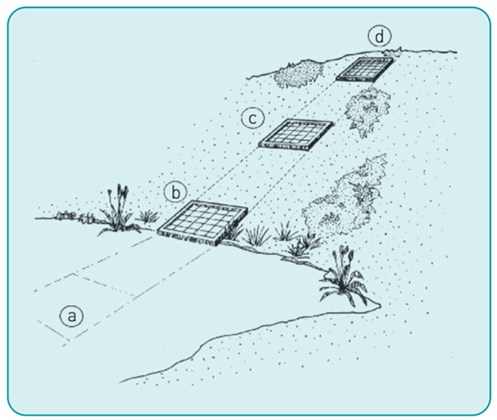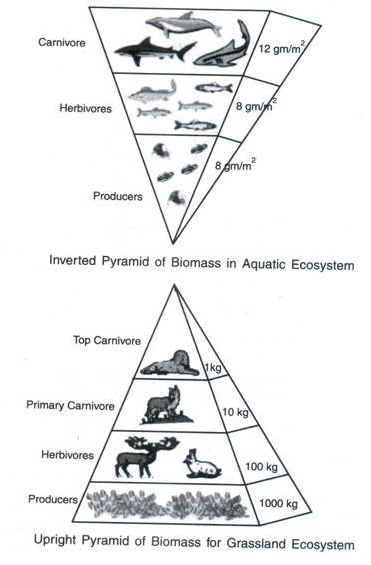# Biomass

## Field Work Techniques

A range of fieldwork techniques can be used to determine the number of organisms in a given area.

1. A __lightmeter __can be used to used to measure the light intensity. This can be useful when looking the things that can affect the rate of photosynthesis.

2. A __quadrat __can be used to count up the number of plants in a square metre. It is a grid that is placed on the ground, and organisms can be counted up based on the type of species that are found. They are placed randomly so that there is no bias, and it is more reliable to compare within different locations.

3. A __transect __can be used to record data at regular intervals. It is a straight line that is placed across a location, and a quadrat will be placed at random intervals along the transect so that the species can be counted up.

﻿## Energy Transfer

Some energy is transferred to less useful forms at each trophic level. This affects the number of organisms at each trophic level, limits the length of a food chain and determines the shape of a pyramid of biomass in an ecosystem.

__Pyramids of biomass __can be used to represent the amount of biomass that can be found at each trophic level in a food chain.

__Biomass is the total mass of the living material that is found in an organism, multiplied by the number of organisms that can be found. __Here you can see that the __producers __(photosynthetic organisms) are at the bottom of the pyramid. As you move up the trophic levels and up the pyramid, the __biomass decreases as energy is wasted. __

This means that less biomass and energy can be passed to the next organisms in the food chain above them. Energy is not completely transferred from one trophic level to another because:

﻿

1. Some food is stored, some food becomes faeces
2. Animals need the glucose to create energy during respiration so that they can survive. This means that they will have less energy to grow.
3. Vertebrates expend energy so that they can keep at a constant yet suitable temperature
4. Carbon dioxide, water, urea, water are some of the materials that are excreted.

## Calculating Efficiency

The percentage efficiency of energy transfer between trophic levels can be calculated using the following equation:

energy transferred to next level ÷ total energy in × 100

E.g. Using the data given, calculate the percentage of biomass passed on from the producer to the primary consumer.Energy transferred to the next level: 8,000 kJ

Total energy: 10,000 kJ

Percentage of biomass from the grass to sheep is: 8,000 / 10,000 x 100 = 80%

Name 3 fieldwork techniques that can be used to study living organisms